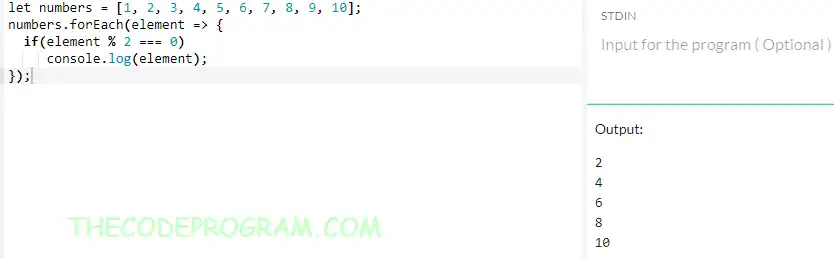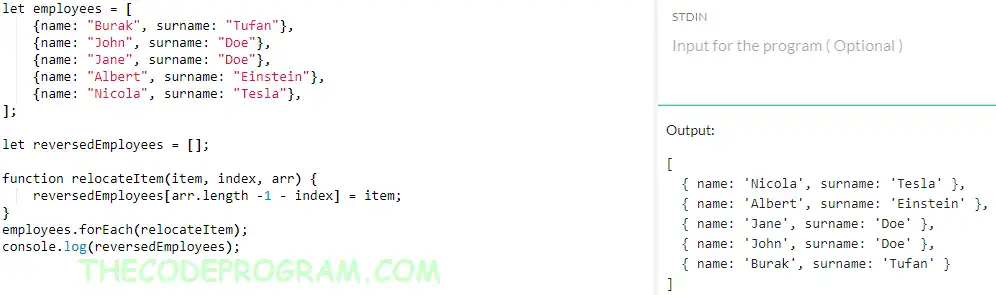## Explanation of forEach function in Javascript

Hello everyone, in this article we are going to talk about foreach method and its usage with Javascript. We will make examples and take a look at performance.

Let's get started.

Fistly, What foes forEach method do
forEach() method iterates a given array and invoke a void function for all elements inside given array.

#### Below you can see the syntaxes of forEach():

##### Arrow function:
``````
let users = ['Burak', 'Hamdi', 'Tufan'];
users.forEach(element => {
//some logic
});
``````
##### Function calling with single parameter
``````
let users = ['Burak', 'Hamdi', 'Tufan'];
function printFnc(el) {
//some logic
}
users.forEach(myFunction);
``````
##### Function calling with all array parameters
``````
let users = ['Burak', 'Hamdi', 'Tufan'];
function printFnc(item, index, arr) {
arr[index] = 'Hello ' + item;
}
function printFnc(el) {
//some logic
}
``````

In this usage, index and arr parameters are optional.

Now let's make some examples about forEach() method.

Below example controls all elements in array and print if even number.

``````
let numbers = [1, 2, 3, 4, 5, 6, 7, 8, 9, 10];
numbers.forEach(element => {
if(element % 2 === 0)
console.log(element);
});
``````
Output will be like below:
``````
2
4
6
8
10
``````Below example you will see an example of forEach method. In this example we handled a JSON object with our declared method. We printed all of objects in aircrafts array.

``````
let aircrafts = [
{brand: "Airbus", model: "A330"},
{brand: "Boeing", model: "777-300ER"},
{brand: "Airbus", model: "A320"},
{brand: "Boeing", model: "737-MAX9"}
];
function printAircraft(el) {
console.log("Aircraft : " + el.brand + " " + el.model);
}
aircrafts.forEach(printAircraft);
``````
Program output will be like below
``````
Aircraft : Airbus A330
Aircraft : Boeing 777-300ER
Aircraft : Airbus A320
Aircraft : Boeing 737-MAX9
``````
Below image yoıu can see the code blockBelow code block we will reverse an array. We will use the array item length and its index with forEach function. In this usage we used the optional index and arr parameters

``````
let employees = [
{name: "Burak", surname: "Tufan"},
{name: "John", surname: "Doe"},
{name: "Jane", surname: "Doe"},
{name: "Albert", surname: "Einstein"},
{name: "Nicola", surname: "Tesla"},
];

let reversedEmployees = [];

function relocateItem(item, index, arr) {
reversedEmployees[arr.length -1 - index] = item;
}
employees.forEach(relocateItem);
console.log(reversedEmployees);
``````
Output will be like below
``````
[
{ name: 'Nicola', surname: 'Tesla' },
{ name: 'Albert', surname: 'Einstein' },
{ name: 'Jane', surname: 'Doe' },
{ name: 'John', surname: 'Doe' },
{ name: 'Burak', surname: 'Tufan' }
]
``````
Below image you can see the usageNow Let's make a comparisation between traditional for loop and forEach method. We will compare their execution times. Below code block you will see the comparisation code.
``````
let total = 0;
const numbers = [85,45,23,96,54,11,55,6524,3941,4,55,66,33,4,5,9,7,17,85,32,455,698,10,85,74,36,41,52,999];
console.log("For Method:" );
console.log("Start : " + window.performance.now());
for (let i = 0; i < numbers.length;i++)
total += numbers[i];
console.log("End : " + window.performance.now());
console.log("ForEach Method:" );
total = 0;
console.log("Start : " + window.performance.now());
numbers.forEach(num => total += num);
var forEachEnd = window.performance.now();
console.log("End : " + window.performance.now());
``````
Below you can see the example output :
``````
For Method:
Start : 301202.7999999523
End : 301202.89999997616

ForEach Method:
Start : 301202.89999997616
End : 301202.89999997616
``````
As you can see foreach a bit more faster than traditional for loop.

Have a good invokes for Each items.

Burak Hamdi TUFAN.

02/04/2022

23/10/2022

19/06/2023